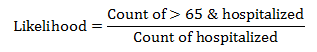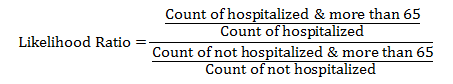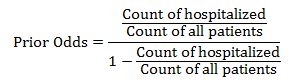# Bivariate Analysis

• Chapter 4 in Big Data in Health Care: Statistical Analysis of Electronic Health Record Read►

# Assignments

Instruction for Submission of Assignments: Assignments should be submitted directly on Blackboard.  In rare situations assignments can be sent directly by email to the instructor. Submission should follow these rules:

A. This problem describes a type of problem typically discussed in Marketing classes, where managers are trained to understand market participation and market share.  We have simplified the number of variables and cases in the problem to make it easier to analyze. A typical realistic problem may have hundreds of variables and thousands of cases. Data►

1. What is the probability of hospitalization given that you are male? Select all males and count the number of patients who were hospitalized. Calculate the probability as the ratio of males hospitalized to number of males.  Video► SWF►
2. Create a contingency table for interaction between age and gender.  Is age independent from gender. Answer►
3. Is insurance independent of age?  Check that the probability of combination of insurance and age can be estimated from the product of probability of insurance and age or use the contingency table of age and insurance.
4. What is the probability associated of being more than 65 years old among hospitalized patients? Start by selecting all hospitalized patients, then count the number among hospitalized patients who are more than 65 years old.  The likelihood or the probability of being over 65 among hospitalized patient is the number of patients hospitalized and above 65 divided by the number of hospitalized patients:5. What is the probability of being hospitalized given you are more than 65 years old?  This time we are switching the condition. Now we are asking for the probability among patients who are more than 65 years old.  So select all patients who are more than 65 years old and then count the number who are hospitalized.  In contrast to the previous question the ratio is calculated by dividing the number of patients above 65 who were hospitalized divided by number above 65 years. Elina's SQL►
6. In predicting hospitalization, what is the likelihood ratio, LR, associated with being more than 65 years old?  This is not the same as the likelihood of being above 65 given that you are hospitalized.  It should be calculated as follows:7. What is the prior odds for hospitalization before any other information is available?  The probability of hospitalization is calculated as the number hospitalized by the number in the sample. Prior odds is calculated as the probability of hospitalization by one minus the probability.  A simpler way to do so, the prior odds is the ratio of number hospitalized divided by the number not hospitalized or as:8. Analyze the data in the Table and report if any two variables are conditionally independent of each other in predicting probability of hospitalization? Consider the pairs  Gender & Age, Age & Insured, and Gender & Insured.  If two events are independent, then the likelihood ratio associated with the combined event should be the product of the likelihood ratios of each event.  If the likelihood ratio cannot be calculated because of division by zero, then skip that check. In using likelihood ratios to test the independence of two variables, note that you have to test it for all levels in the variable.  So for example, if we are examining the independence of age and gender, then you would test the independence of four set of combination of variables from their components:
• Likelihood ratio Age>65 and Male = Likelihood ratio of Age>65 * Likelihood ratio of Male
• Likelihood ratio Age>65 and Female = Likelihood ratio of Age>65 * Likelihood ratio of Female
• Likelihood ratio Age<=65 and Male = Likelihood ratio of Age<=65 * Likelihood ratio of Male
• Likelihood ratio Age<=65 and Female = Likelihood ratio of Age<=65 * Likelihood ratio of Female
Keep in mind that because the number of cases are too few, many ratios cannot be calculated.   Video► SWF►

# More

 Copyright © 1996 Farrokh Alemi, Ph.D. Most recent revision 09/20/2020.  This page is part of the course on Statistical Process Control, this is the lecture on Introduction.